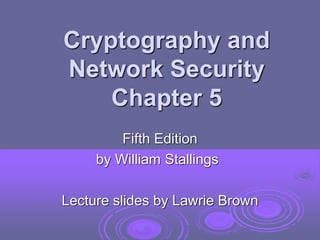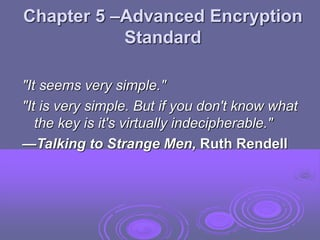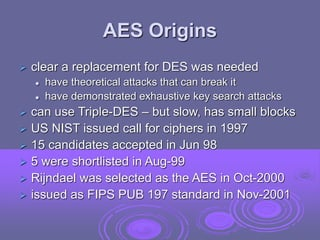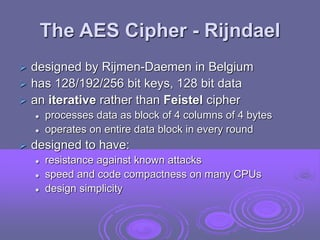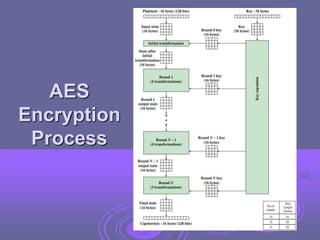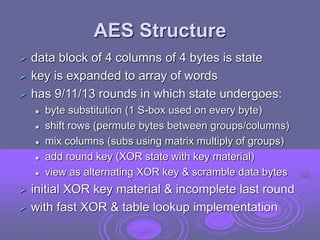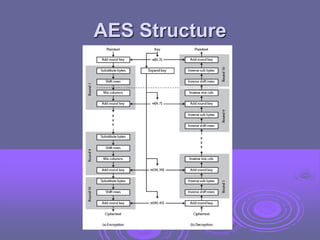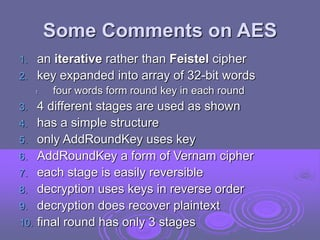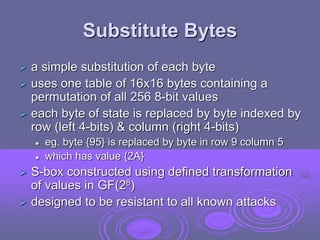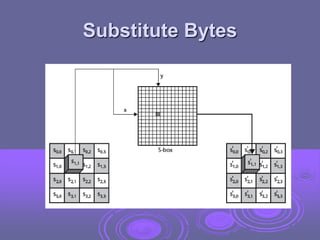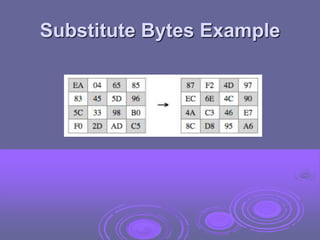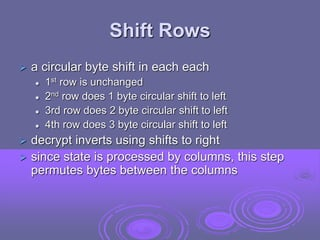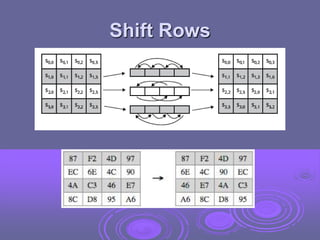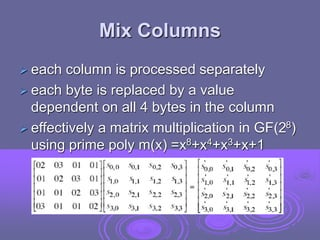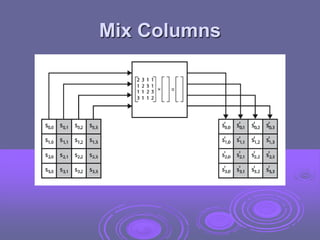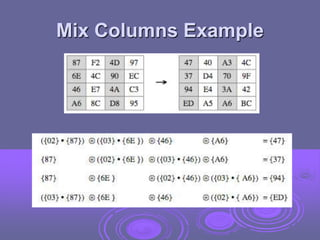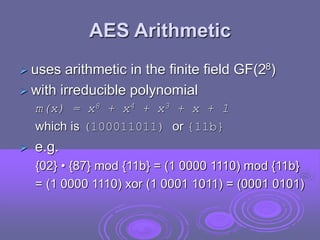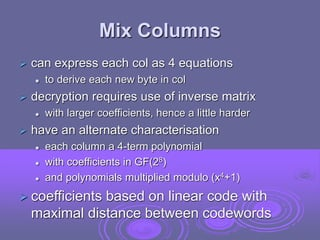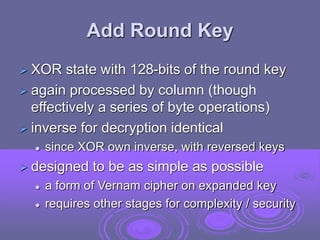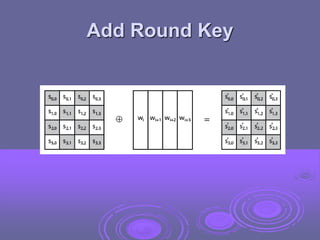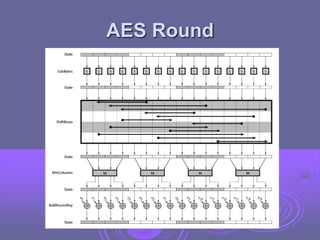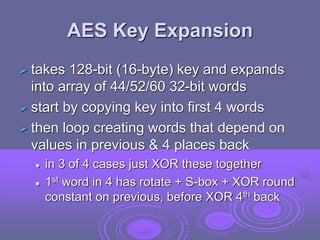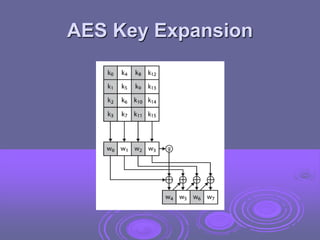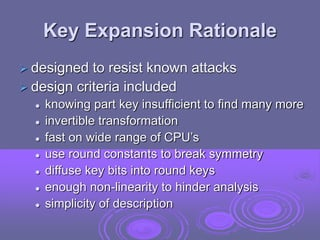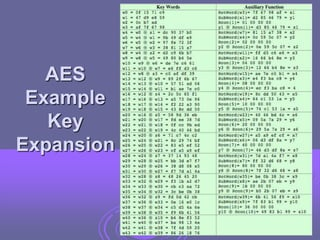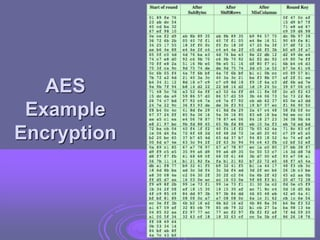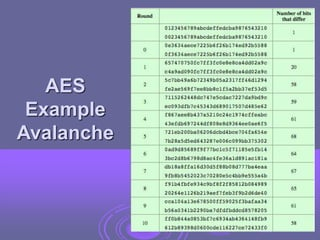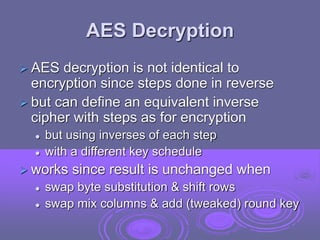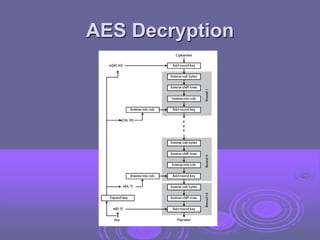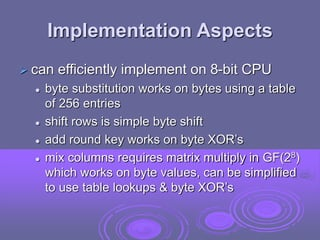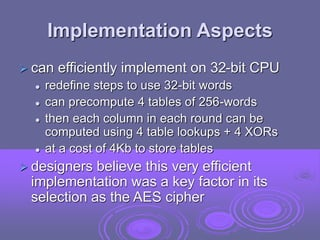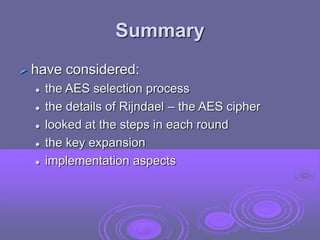1 of 32

### CR 05 - Advanced Encryption Standard.ppt

1. Cryptography and Network Security Chapter 5 Fifth Edition by William Stallings Lecture slides by Lawrie Brown
2. Chapter 5 –Advanced Encryption Standard "It seems very simple." "It is very simple. But if you don't know what the key is it's virtually indecipherable." —Talking to Strange Men, Ruth Rendell
3. AES Origins  clear a replacement for DES was needed  have theoretical attacks that can break it  have demonstrated exhaustive key search attacks  can use Triple-DES – but slow, has small blocks  US NIST issued call for ciphers in 1997  15 candidates accepted in Jun 98  5 were shortlisted in Aug-99  Rijndael was selected as the AES in Oct-2000  issued as FIPS PUB 197 standard in Nov-2001
4. The AES Cipher - Rijndael  designed by Rijmen-Daemen in Belgium  has 128/192/256 bit keys, 128 bit data  an iterative rather than Feistel cipher  processes data as block of 4 columns of 4 bytes  operates on entire data block in every round  designed to have:  resistance against known attacks  speed and code compactness on many CPUs  design simplicity
5. AES Encryption Process
6. AES Structure  data block of 4 columns of 4 bytes is state  key is expanded to array of words  has 9/11/13 rounds in which state undergoes:  byte substitution (1 S-box used on every byte)  shift rows (permute bytes between groups/columns)  mix columns (subs using matrix multiply of groups)  add round key (XOR state with key material)  view as alternating XOR key & scramble data bytes  initial XOR key material & incomplete last round  with fast XOR & table lookup implementation
7. AES Structure
8. Some Comments on AES 1. an iterative rather than Feistel cipher 2. key expanded into array of 32-bit words 1. four words form round key in each round 3. 4 different stages are used as shown 4. has a simple structure 5. only AddRoundKey uses key 6. AddRoundKey a form of Vernam cipher 7. each stage is easily reversible 8. decryption uses keys in reverse order 9. decryption does recover plaintext 10. final round has only 3 stages
9. Substitute Bytes  a simple substitution of each byte  uses one table of 16x16 bytes containing a permutation of all 256 8-bit values  each byte of state is replaced by byte indexed by row (left 4-bits) & column (right 4-bits)  eg. byte {95} is replaced by byte in row 9 column 5  which has value {2A}  S-box constructed using defined transformation of values in GF(28)  designed to be resistant to all known attacks
10. Substitute Bytes
11. Substitute Bytes Example
12. Shift Rows  a circular byte shift in each each  1st row is unchanged  2nd row does 1 byte circular shift to left  3rd row does 2 byte circular shift to left  4th row does 3 byte circular shift to left  decrypt inverts using shifts to right  since state is processed by columns, this step permutes bytes between the columns
13. Shift Rows
14. Mix Columns  each column is processed separately  each byte is replaced by a value dependent on all 4 bytes in the column  effectively a matrix multiplication in GF(28) using prime poly m(x) =x8+x4+x3+x+1
15. Mix Columns
16. Mix Columns Example
17. AES Arithmetic  uses arithmetic in the finite field GF(28)  with irreducible polynomial m(x) = x8 + x4 + x3 + x + 1 which is (100011011) or {11b}  e.g. {02} • {87} mod {11b} = (1 0000 1110) mod {11b} = (1 0000 1110) xor (1 0001 1011) = (0001 0101)
18. Mix Columns  can express each col as 4 equations  to derive each new byte in col  decryption requires use of inverse matrix  with larger coefficients, hence a little harder  have an alternate characterisation  each column a 4-term polynomial  with coefficients in GF(28)  and polynomials multiplied modulo (x4+1)  coefficients based on linear code with maximal distance between codewords
19. Add Round Key  XOR state with 128-bits of the round key  again processed by column (though effectively a series of byte operations)  inverse for decryption identical  since XOR own inverse, with reversed keys  designed to be as simple as possible  a form of Vernam cipher on expanded key  requires other stages for complexity / security